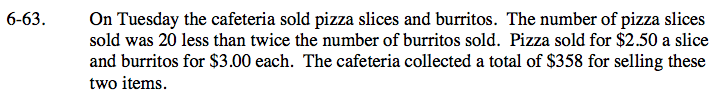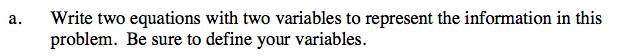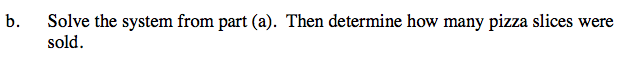### Home > CAAC > Chapter 6 > Lesson 6.2.3 > Problem6-63

6-63.
1. On Tuesday the cafeteria sold pizza slices and burritos. The number of pizza slices sold was 20 less than twice the number of burritos sold. Pizza sold for $2.50 a slice and burritos for$3.00 each. The cafeteria collected a total of \$358 for selling these two items. 6-63 HW eTool (Desmos). Homework Help ✎

1. Write two equations with two variables to represent the information in this problem. Be sure to define your variables.

2. Solve the system from part (a). Then determine how many pizza slices were sold.p = pizza slices (#)
b = burritos (#)

One equation should be about the amount of money collected from the burritos and pizza slices sold.
The other equation should be about the number of pizza slices sold compared to the number of burritos sold.

2.50p + 3b = 358
p = 2b − 20Use the substitution method with the equations you found in part (a).